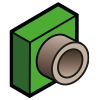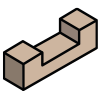# Line-based construction (Water Overlay)

Line based constructions are constructions for which their geometry is interpreted as a 2 dimensional line with two end points.

Examples of line based constructions are the following hydraulic structures:

Icon Name NameCulvert Flow in both directions possible.Weir Conditional flow, based on the weir's height. Flow possible in both ways.Pump Pumps water from the entry with the lowest water level to the entry with the highest water level.

## Polygon skeleton

A method to obtain a line geometries from a polygonal geometry is called topological skeletonization. The method used by Tygron Platform is described in: Polygon skeleton. In the case of Line-based constructions, we want to obtain only a single line segment. Currently only a simple implementation is used:

1. Skeletonize a construction's polygon. This potentially produces a multi-line geometry.
2. Take the first point and the last point of this line geometry. These two points will form the line segment used for the line-based construction.

The method above is simple, but not robust in all cases. See the following images:

In case one, the resulting skeleton lines have 3 different end points. Which of these points will be the first and last end point is dependent on the sequence of coordinates in the polygon.
In case two, the polygon is a perfect circle, resulting in no skeleton line.
In case three, the skeleton line is very small, for example 1 centimeter. This may not be desirable when the end points need to be separated more than a certain amount.

More complex but common situation:

Generally, this kind of polygon is undesirable for a line-based construction, since it will generate a skeleton with many end points.